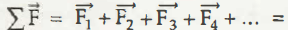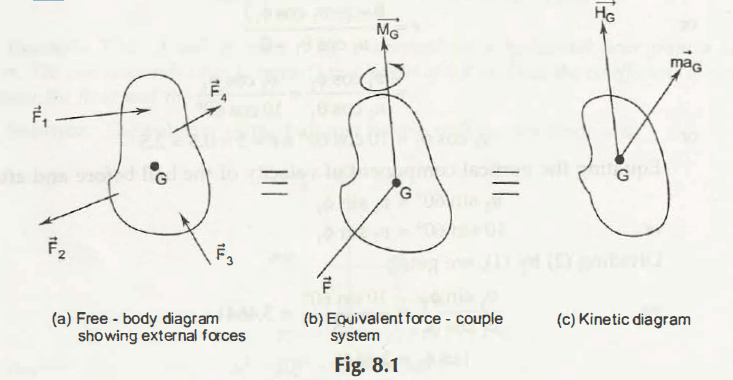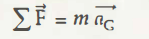According to the newton’s second Law of motion F = ma

This also expresses that if forces are applied and act on a rigid body, then

Angular momentum of its centre of the gravity = Acceleration of G or the center of the body.

It means if the different forces are applied on the rigid body then their sum will be the resultant of all external forces. It meanstill the end magnitude of the force in your equation

and thus LetResultant of all forces those act on a body.

Now, what are the conditions of forces those act on a rigid body? To get its proper answer look at the following figures.In the figure (a), The different forces F1, F2, F3 and F4 act and the different forces are some external forces. In the figure (b) Equivalent force shows the couple system and in the last figure you can get that kinetic forces act. Now, the G is the center of gravity. HG represents the angular momentum of the center of the gravity.

HG = Angular momentum of the body having G is the centre of Gravity = Acceleration of the body with the mass centre G

According to the second law of Newton,

F = ma

Here,Where, m is the body mass.

An important concept here is the summation of moments about the mass centre = time rate of change of the angular momentum

Now, it is clear that the working of the external forces can be expressed by the next figure as couple system G. In addition, by using equation from the figure (a) and using equation from the figure (b), the Kinetics digram figure c is derived.

Now, it is clear that how force mass and acceleration are related to each other and how gravity plays an important role in finding out the relation.

Links of Next Mechanical Engineering Topics:-### Customer Reviews

My Homework Help
Rated 5.0 out of 5 based on 510 customer reviews at